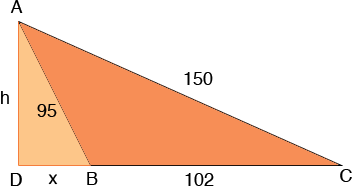SEARCH HOMEMath Central Quandaries & QueriesQuestion from fhay, a student: In an oblique triangle, If side a=95, side b=102 and side c = 150 find the missing angles solved by right angles...Thank's a lot......Hi Fhay,

I drew the triangle and extended the side of length 102 units to form a right triangle ADC.Since triangle ADB is a right triangle you can use Pythagoras' Theorem to find a relationship between h and x. Also triangle ADC is a right triangle so again using Pythagoras' Theorem you can find a second relationship between x and h. Solve these two equations for x and h.

Now you can use trigonometry to find the angles. For example the sine of the angle BCA is h/150.

I hope this helps,
PennyMath Central is supported by the University of Regina and The Pacific Institute for the Mathematical Sciences.